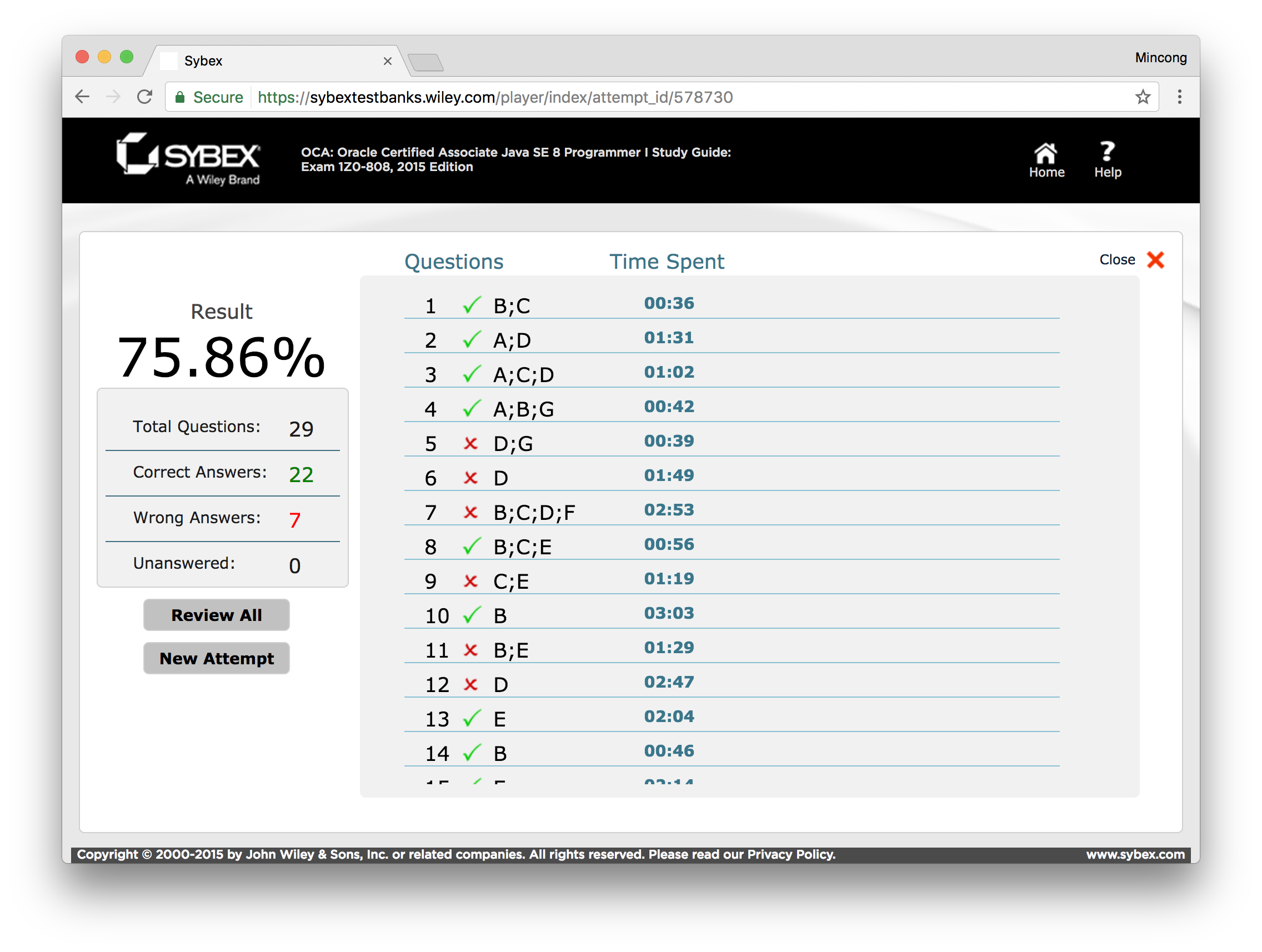Today, I’m going to review my online test, chapter 4 of Oracle Certified Associate (OCA), provided by SYBEX. If you need to access to this online resources, you need to buy their book first. See https://sybextestbanks.wiley.com/.## Question 5

Given the following method, which of the method calls return `2`? (Choose all that apply)

1. `howMany();`
2. `howMany(true);`
3. `howMany(true, true);`
4. `howMany(true, true, true);`
5. `howMany(true, {true});`
6. `howMany(true, {true, true});`
7. `howMany(true, new boolean);`

The answer is DG. The answer A is wrong because it does not match the types of input parameters, the code does not compile. The answer B is wrong because it creates a vararg of size 0. The answer C returns 1. The answer D return 2 which is correct. The answer E and F do not compile because they do not declare an array properly, e.g. it should be `new boolean[] {true}` for answer E. The answer G is correct, which creates an empty array with 2 elements. The tricky thing here is the “Anonymous Array” as `{ ... }` without the word `new`. This is anonymous because you don’t specify the type and size. However, it is only allowed in initialization as the following:

## Question 6

Which of the following are true? (choose all that apply)

1. Package private access is more lenient than protected access.
2. A `public` class that has private fields and package private methods is not visible to classes outside the package.
3. You can use access modifiers so only some of the classes in a package see a particular package private class.
4. You can use access modifiers to allow read access to all methods, but not any instance variable.
5. You can use access modifiers to restrict read access to all classes that begin with the word `Test`.

The answer is D. The answer A is wrong because package private access is less lenient than protected access: package private means that only classes within the same package call use this method, while protected access means that classes within the same package, or classes extended the target class can see this method. The answer B is wrong, because a public class is visible outside of the package regardless the visibility of its methods. The answer C is wrong, because package private access applies to the whole package. The answer E is wrong because Java does not have such capability. The answer D is correct, this approach is used for all the immutable classes.

## Question 7

Given the following `my.school.Classroom` and `my.city.School` class definitions, which line numbers in `main()` generate a compiler error? (Choose all that apply)

1. None, the code compiles fine.
2. Line 8
3. Line 9
4. Line 10
5. Line 11
6. Line 12

The answer is BCDF. The answer B is wrong because `globalKey` has package private access, the main method cannot see this variable. The answer C is wrong for the same reason, the constructor is package private, so it is not visible for the main method. The answer D is private, so invisible for main method. The answer E is correct because `floor` is public. The answer E is incorrect because teach’s name is protected, only sub-classes of classroom, or any class in the same package can see this variable.

## Question 12

What is the output of the following code?

1. `02`
2. `08`
3. `2`
4. `8`
5. The code does not compile.
6. An exception is thrown.

The answer is D. The class `RopeSwing` has an instance initializer and not a static initializer. However, the class `RopeSwing` is never constructed, so the method `System.out.println(repo1.length);` is never called. Since the length is a static variable, repo 1 and repo 2 update the same variable.

## Question 17

Which of the following are output by the following code? (Choose all that apply)

1. `s1 = a`
2. `s1 = s1`
3. `s2 = s2`
4. `s2 = s2b`
5. `s3 = a`
6. `s3 = null`
7. The code does not compile.

The answer is BDE. Since Java is pass-by-value, assigning a new object to `a` des not change the caller, so B is correct. However, calling the method of the same reference does affect the caller, so D is correct. Finally, the method `work` returns a reference of string builder containing a character “a”, so E is correct.